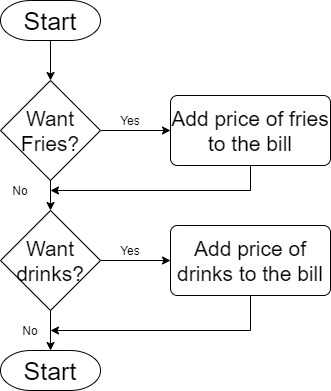Let’s learn programming concepts and Scratch practicals and master them. In this article, you can learn the basic control structures on flow charts and learn how to build a Scratch code for a given flow chart with selection and repetition. Here I have discussed the following topics in your grade 9 programming lesson. You can easily navigate through the article with the table of content given below.

We recommend starting with simple flowcharts and coding with Scratch. You can refer to our Simple Flowcharts with Scratch codes article to learn more.

## Selection

Selection Logic (control structure) allows continuing the flow in two different paths according to the condition. If the condition is Yes (true) then it goes on one side, otherwise, it goes on another side. You can imagine that you are in a junction and you must decide the direction. You ask the following question from yourself.

Is the red color side leads to my destination?

If the answer to your question is yes, then you select to go towards the left side. Otherwise, you go to the right side. Here the condition is the question that you asked from yourself. Likewise, in flowcharts, you can have conditions.

There are two types of selections we can identify in flow charts. The first one is a simple selection, where it has only one selection statement. The above flowchart is an example of a simple selection.

In multiple selection, we use multiple selection statements in a single flowchart. Let’s write the algorithm of a scenario that we went to a cafeteria and order foods and drink.

• If you want fries then add it to the bill
• If you want drinks then add it to the bill

There are two decision boxes to indicate the two selections.## Repetition

Repetition of the process is called an “iteration”. Repetition allows for a portion of an algorithm or computer program to be executed any number of times dependent on some condition being met. An occurrence of repetition is usually known as a loop. The condition allows us to determine the number of loops.

When we need to print numbers from 1 to 100, it is easy when we use repetition. We can mention a starting value and then increase the value by one each time inside the loop as shown below.You can build a Scratch program to this flowchart as well.Let’s discuss more on the flow chart. Here we print the values one by one. The initial value of the number is 1. Then it goes through a decision box. We check a condition whether the number is less than 101. If it is yes then we print the number and add 1 to the number. It displays as number = number +1. This is not the equal sign that you learn in mathematics. We call this as an assignment operator.  Therefore it symbols that we add 1 to the current value of the number. This process and output “Print number” iterate 100 times until all the numbers print.  Then it end as the condition become false due to the value of the number is 101.